# GMAT Math : DSQ: Calculating the length of an edge of a cube

## Example Questions

### Example Question #1 : Cubes

What is the length of the edge of a cube?

1) Its volume is 1,728 cubic meters.

2) Its surface area is 864 square meters

Statement 2 ALONE is sufficient, but Statement 1 alone is not sufficient.

Statement 1 ALONE is sufficient, but Statement 2 alone is not sufficient.

Statements 1 and 2 TOGETHER are not sufficient.

EACH statement ALONE is sufficient.

BOTH statements TOGETHER are sufficient, but neither statement ALONE is sufficient.

EACH statement ALONE is sufficient.

Explanation:

Call the sidelength, surface area, and volume of the cube,, and, respectively.

Then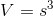or, equivalently,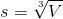So, given statement 1 alone - that is, given only the volume, you can demonstrate the sidelength to be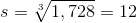Also,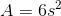or, equivalently,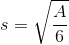Given statement 2 alone - that is, given only the surface area, you can demonstrate the sidelength to be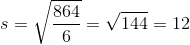Therefore, the answer is that either statement alone is sufficient.

### Example Question #212 : Geometry

A sphere is inscribed inside a cube. What is the volume of the sphere?

Statement 1: The surface area of the cube is 216.

Statement 2: The volume of the cube is 216.

EITHER statement ALONE is sufficient to answer the question.

BOTH statements TOGETHER are sufficient to answer the question, but NEITHER statement ALONE is sufficient to answer the question.

Statement 2 ALONE is sufficient to answer the question, but Statement 1 ALONE is NOT sufficient to answer the question.

Statement 1 ALONE is sufficient to answer the question, but Statement 2 ALONE is NOT sufficient to answer the question.

BOTH statements TOGETHER are insufficient to answer the question.

EITHER statement ALONE is sufficient to answer the question.

Explanation:

The diameter of a sphere inscribed inside a cube is equal to the length of one of the edges of a cube. From either the surface area or the volume of a cube, the appropriate formula can be used to calculate this length. Half this is the radius, from which the formula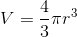can be used to find the volume of the sphere.

### Example Question #1 : Cubes

What is the length of edge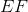of cube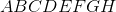(1)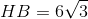.

(2)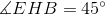.

Statement 2 alone is sufficient.

Statement 1 alone is sufficient.

Statements 1 and 2 together are not sufficient.

Each statement alone is sufficient.

Both statements together are sufficient.

Statement 1 alone is sufficient.

Explanation:

In order to find the length of an edge, we would need any information about one of the faces of the cube or about the diagonal of the cube.

Statement 1 gives us the length of the diagonal of the cube, since the formula for the diagonal is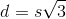whereis the length of an edge of the cube andis the length of the diagonal we are able to find the length of the edge. Therefore statement 1 alone is sufficient.

Statement 2 alones is insufficient, it gives us something we can already tell knowing that ABCDEFGH is a cube.

Statement 1 alone is sufficient.

### Example Question #2 : Cubesis a cube. What is the length of edge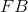?

(1) The volume of the cube is.

(2) The area of faceis.

Statements 1 and 2 together are not sufficient.

Statement 2 alone is sufficient.

Statement 1 alone is sufficient.

Both statements together are sufficient.

Each statement alone is sufficient.

Each statement alone is sufficient.

Explanation:

Like we have previously seen, to find the length of an edge, we need to have information about the other faces or anything else within the cube.

Statement 1 tells us that the volume of the cube is, from this we can find the length of the side of the cube. Statement 1 alone is sufficient.

Statement 2, tells us that the area of ABCD is, similarily, by taking the square root of this number, we can find the length of the edge of the cube.

Therefore each statement alone is sufficient.

### Example Question #3 : Cubes

Find the length of an edge of the cube.

1. The volume of the cube is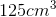.
2. The surface area of the cube is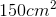.

Each statement alone is sufficient to answer the question.

Statements 1 and 2 are not sufficient, and additional data is needed to answer the question.

Statement 1 alone is sufficient, but statement 2 alone is not sufficient to answer the question.

Statement 2 alone is sufficient, but statement 1 alone is not sufficient to answer the question.

Both statements taken together are sufficient to answer the question, but neither statement alone is sufficient.

Each statement alone is sufficient to answer the question.

Explanation:

Statement 1:  Use the volume formula for a cube to solve for the side length.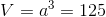whererepresents the length of the edge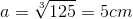Statement 2:   Use the surface area formula for a cube to solve for the side length.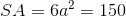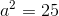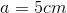Each statement alone is sufficient to answer the question.

Tired of practice problems?

Try live online GMAT prep today.